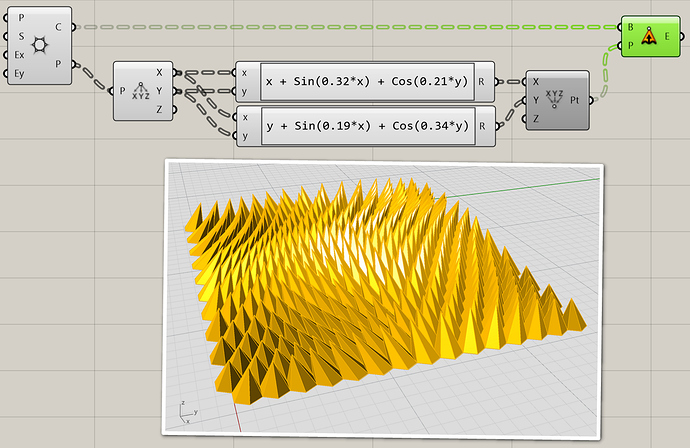# Extruded Hexagonal cone series

Hello, I am working on a computational modeling project, and am having issues when it comes to pulling cones in different directions to achieve the look that I am supposed to mock. The base surface is completely flat so the only reason you see the wave effect is based on the direction/angle that each individual cone is standing (I would assume based off of attractor points of some sort, which is where I get confused as how to make). any feedback would be appreciated!

1 Like

You’ll have to decide for yourself how you want to get this done. Equations? The UVs of a surface? Attractor points? Attractor lines? Fields? You have a lot of option but you need to decide how you want to control this before anyone can help you figure out the details.

How is this thread different from this one by @Snuttal1 ? Are you both taking the same class?

1 Like

we are in the same class, but there was a lack of response to the later question on his, so I decided to start a new thread describing the issue.

This response works most closely to what I intended the outcome would be; however, the only concern is that the way the cones are pointing are not in differentiating linear directions. I am just unsure how to do that.1 Like

I would like to use attractor lines

You could change the equations for example. Instead of having completely separate equations for x and y, you could instead generate a single strength value for every (x,y) pair, and then multiple that factor with a direction vector. This would guarantee that all your cones are deforming along a single direction only.

And here’s a way to deform a pointcloud using various lines. The distance from each point to all lines is mapped onto a falloff curve and then multiplied by the line tangent vector. This results in n vector forces on each point (one for each line) and these are summed together to yield the final deformation.

line deform.gh (14.2 KB)

Thank you all